Function Repository Resource:

# DualNumber

Represent a dual number

Contributed by: Jan Mangaldan
 ResourceFunction["DualNumber"][a,b] returns the dual number a+bε.

## Details

Dual numbers are hypercomplex numbers of the form a+bε, where a and b are real numbers and ε satisfies the relation ε2=0.
Dual numbers work much like complex numbers.
Dual numbers are algebraically identical to the field of algebraic infinitesimals. They can be used in the field of nonstandard analysis.
Because of the similarities between dual numbers and complex numbers, this function imitates the Wolfram Languages treatment of complex numbers in many ways.
To provide a clear distinction between dual numbers and complex numbers, all dual numbers should be entered using the form ResourceFunction["DualNumber"][a,b], where a and b are real numbers.

## Examples

### Basic Examples (3)

Get the dual number 1+2ε:

 In:=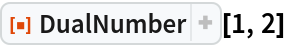Out=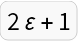In:=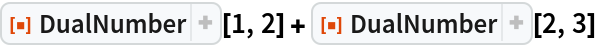Out=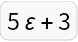Multiply two dual numbers:

 In:=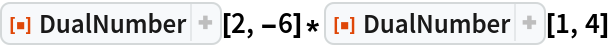Out=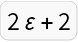### Applications (5)

A procedurally-defined function:

 In:=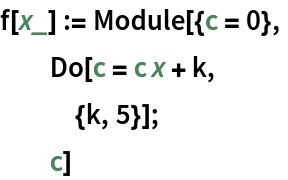Evaluate at a dual number argument with unit dual part:

 In:=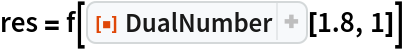Out=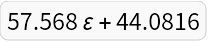The real part is equal to the function evaluated at the real part of the argument:

 In:=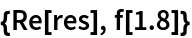Out=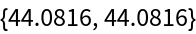The dual part is equal to the derivative of the function:

 In:=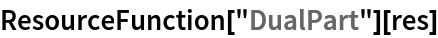Out=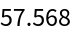Compare with a central difference approximation:

 In:=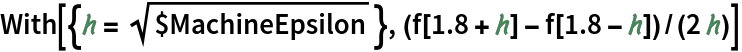Out=### Properties and Relations (4)

In the conjugate of a dual number, the sign of the dual part is reversed:

 In:=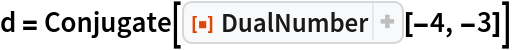Out=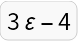Use Re and the resource function DualPart to extract the real and dual parts of a dual number:

 In:=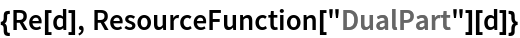Out=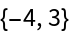The sign of a dual number is defined to be the sign of its real part:

 In:=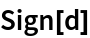Out=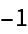The absolute value of a dual number is the absolute value of its real part:

 In:=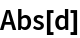Out=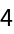Dual numbers have the following "polar form":

 In:=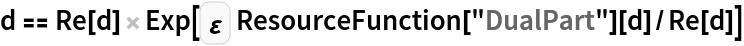Out=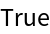Use Norm to get the standard Euclidean length of a dual number:

 In:=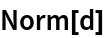Out=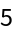Evaluate the exponential of a dual number:

 In:=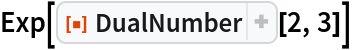Out=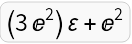Raise a dual number to a dual number power:

 In:=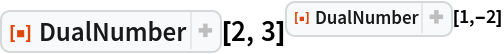Out=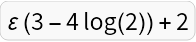Just as with complex numbers, Mod works on dual numbers:

 In:=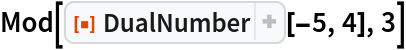Out=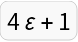## Version History

• 1.0.0 – 05 February 2021

## Author Notes

The implementation is adapted from this Mathematica Stack Exchange post: https://mathematica.stackexchange.com/a/13926